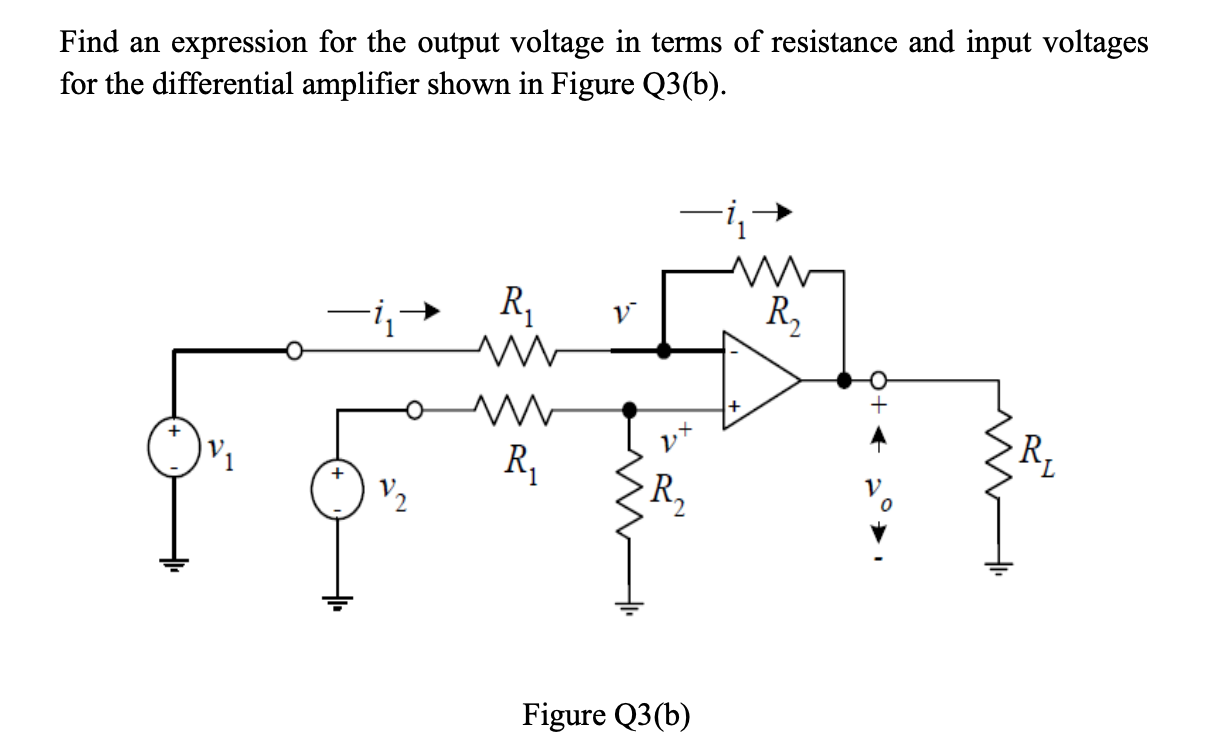# Question Solved1 AnswerFind an expression for the output voltage in terms of resistance and input voltages for the differential amplifier shown in Figure Q3(b). in- M R R, M R R, V2 R, W Figure Q3(b)SJPREG The Asker · Electrical EngineeringTranscribed Image Text: Find an expression for the output voltage in terms of resistance and input voltages for the differential amplifier shown in Figure Q3(b). in- M R R, M R R, V2 R, W Figure Q3(b)
More
Transcribed Image Text: Find an expression for the output voltage in terms of resistance and input voltages for the differential amplifier shown in Figure Q3(b). in- M R R, M R R, V2 R, W Figure Q3(b)